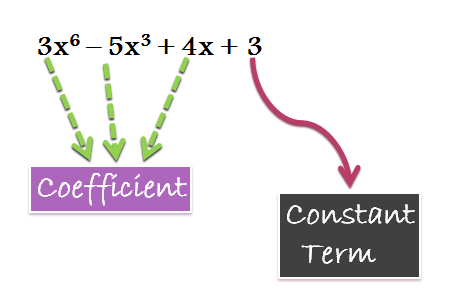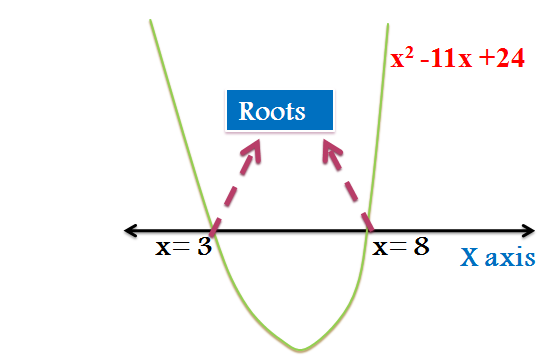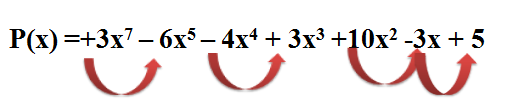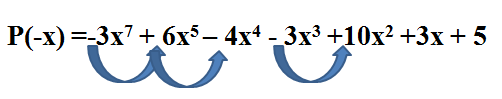# Algebra

#### FUNCTIONS-1

Theory of Equations: 1

Theory of Equations:

This lesson is the starting point of Algebra and deals with some very fundamental properties and theorems related to algebraic functions. So, we’ll study all such theorems and concepts in detail thereby to lay the foundation of algebra.

Polynomial:

Polynomial is derived from two words- poly meaning many and nomial means terms, so polynomial can be said to an algebraic expression involving many terms. Here one can witness the variables along with their exponents, their coefficients, mathematical operators like plus and minus and a constant term (not necessary). The polynomial function can be of a single variable or multiple variables.

e.g.Degree of a Polynomial:

The highest exponent of the variable in a polynomial is known as the degree of the polynomial. Eg. The degree of 4x5 -3x +2 is 5. Similarly the degree of  5x4 – 3x3 +x  is 4 .

Roots of a Polynomial Equation:

The roots of a polynomial, are the values of the variable that make it zero. In other words, the roots are those values of the variable at which the value of the polynomial becomes zero. It can also be said that roots are those value of x (if it is a single variable in x) at which the curve of the function cuts the X axis.

For example:  Consider a polynomial x2 – 11x + 24. This polynomial can be factorised as: x2 – 11x + 24 = (x-3)(x-8)

If we put x = 3 or x = 8, then the value of the polynomial becomes zero or we can say that x =3 and x=8 are the roots.Note:  A polynomial equation of nth degree has n roots.

For example p(x) = 3x7 – 4x6 + 5x5 +4x – 11 is a polynomial equation of degree 7, so it will have 7 roots. Some roots may be real while some may be complex

Remainder Theorem:

If a polynomial f(x) is divided by (x-a), then the remainder obtained on such division is f(a).

Here f(x) is the dividend, (x-a) is the divisor, let q(x) be the quotient obtained and remainder be r.

So Dividend = Divisor*Quotient + Remainder

f(x) = (x-a)q(x) + r

Put x = a , we get

f(a) = r

Thus, the remainder obtained is equal to f(a).

Factor Theorem:

It is an extension of Remainder theorem. If the remainder obtained is zero or f(a) is equal to zero, then (x-a) is a factor of f(x).Polynomial of nth degree :

A polynomial of nth degree can be written as:Example 5: If the roots of the equation x3 -14x2 -84x +216 are in geometrical progression, then find the three roots.

Solution: Let the three roots be a/r , a and ar of the given equation.

Product of the roots = (a/r)*a*(ar) = -216  or a = -6

Thus, the roots are -6/r , -6 and -6r

Sum of the roots = -6/r + -6+ -6r = 14

6/r +6r = -20

3/r +3r = -10

3r2 +10r +3 = 0

r = -3 or -1/3

Thus, the three roots are 2, -6 , 18Example 6: Solve the equation: x4 -4x3 + 5x2 -2x -2 =0 , given that one root is 1-i.

Solution: Given that one root is 1-i, so by conjugate pairs theorem, 1+i should also be a root. Also, as the degree of the polynomial is 4, there are exactly four roots.

Let the other two roots be α and β

α+ β + 1 + i + 1-i = 4

α+β = 2

Also product of roots = αβ(1-i)(1+i) = -2  or αβ = -1

α(2-α) = -1 or 2α-α2 = -1

α,β= 1±√2

Thus the four roots are 1-i , 1+i , 1+√2 and 1-√2

Descarte’s Rule of Sign:

This theorem helps us in estimating the number of positive, negative and conjugate roots of a polynomial equation. Let us understand it with an example

P(x) = 3x7 – 6x5 – 4x4 + 3x3 +10x2 -3x + 5

The number of changes in the sign of consecutive terms will give the maximum number of positive roots.There are 4 changes in the sign, so maximum number of positive roots =4

For maximum number of negative roots, find P(-x) and calculate the number of changes in the sign of the consecutive terms.Thus, the maximum number of negative roots = 3

Also, we know that

Total Roots = Positive Roots + Negative Roots + Complex Roots

The change in sign gives the maximum number of positive /negative roots. Also we know that the complex roots occur in pair.

Number of positive roots in this case  is either 4, 2 or 0

Number of negative roots = 3 or 1

Complex roots = 0 or 2 or 4 or 6

In a polynomial with real coefficients, the number of positive roots is either equal to the number of changes in sign of P(x) or less than that by an even number. Similarly the number of negative roots of the equation is either equal to the number of changes in the sign of consecutive terms of P(-x) or less than that by an even number.

Example 7: Consider the equation : 4x3 -6x2 -3x + 2 =0. Discuss the nature of roots- positive negative and complex.

Solution : P(x) = 4x3 -6x2 -3x + 2= 0

The total number of roots = 3 as the degree of the polynomial is 3.

For positive Roots

P(x) = 4x3 -6x2 -3x + 2= 0

Number of sign changes = 2

So, number of positive roots = 2 or 0

For Negative Roots

P(-x) = - 4x3 -6x2 +3x + 2= 0

Number of sign changes = 1

So, there is atleast one negative root

Complex Roots = 0 or 2.

 Positive Roots Negative Roots Complex Roots 2 1 0 0 1 2

Example 8 : Discuss the nature of the roots of the equation:

4x4 -5x3 -2x2 -6x +1 =0

Solution: Let P(x) = 4x4 -5x3 -2x2 -6x +1 =0

As the degree is 4, there are a total of 4 roots.

For Positive Roots:

P(x) = 4x4 -5x3 -2x2 -6x +1 =0

Number of sign changes = 2

Number of positive roots = 2 or 0

For Negative Roots:

P(-x) =  4x4 +5x3 -2x2 +6x +1 =0

Number of sign changes = 2

Number of negative roots = 2 or 0

 Positive Roots Negative Roots Complex Roots 2 0 2 2 2 0 0 0 4 0 2 2